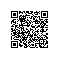# 动态规划

Given a string S, find the longest palindromic substring in S. You may assume that the maximum length of S is 1000, and there exists one unique longest palindromic substring.

• dp[i][j] 表示子串s[i…j]是否是回文
• 初始化：dp[i][i] = true (0 <= i <= n-1);  dp[i][i-1] = true (1 <= i <= n-1); 其余的初始化为false
• dp[i][j] = (s[i] == s[j] && dp[i+1][j-1] == true)

C++实现代码：

#include<iostream>
#include<string>
#include<cstring>
using namespace std;

class Solution
{
public:
string longestPalindrome(string s)
{
if(s.empty())
return NULL;
int start=0;
int end=0;
int n=s.length();
bool dp[n][n];
memset(dp,false,sizeof(dp));
int i;
dp=true;
for(i=1;i<n;i++)
{
dp[i][i]=true;
dp[i][i-1]=true;
}
int k;//k用于记录从i开始的子串的长度,当长度为1是肯定是回文，从len=2开始判断
for(k=2;k<=n;k++)
{
for(i=0;i<=n-k;i++)
{
if(s[i]==s[i+k-1]&&dp[i+1][i+k-2])
{
dp[i][i+k-1]=true;
if(k>end-start+1)
{
start=i;
end=i+k-1;
}
}
}
}
return s.substr(start,end-start+1);
}
};

int main()
{
string s1="bbb";
Solution s;
cout<<s.longestPalindrome(s1)<<endl;
}

http://www.leetcode.com/2011/11/longest-palindromic-substring-part-ii.html

T = # a # b # a # a # b # a #
P = 0 1 0 3 0 1 6 1 0 3 0 1 0C表示当前的回文中心，L和R处的线表示以C为中心可以到达的最左和最右位置，如果知道这些，我们如何可以更好的计算C后面的P[i].Now we are at index i = 15, and its mirrored index around C is i’ = 7. Is P[ 15 ] = P[ 7 ] = 7?if P[ i' ] ≤ R – i,
then P[ i ] ← P[ i' ]
else P[ i ] ≥R – i. (这里下一步操作是扩充 P[ i ].

C++实现代码：

#include<iostream>
#include<string>
#include<cstring>
#include<new>
using namespace std;

class Solution
{
public:
// Transform S into T.
// For example, S = "abba", T = "^#a#b#b#a#$". // ^ and$ signs are sentinels appended to each end to avoid bounds checking
string preProcess(string s)
{
int n = s.length();
if (n == 0) return "^$"; string ret = "^"; for (int i = 0; i < n; i++) ret += "#" + s.substr(i, 1); ret += "#$";
return ret;
}

string longestPalindrome(string s)
{
string T = preProcess(s);
int n = T.length();
int *P = new int[n];
int C = 0, R = 0;
for (int i = 1; i < n-1; i++)
{
int i_mirror = 2*C-i; // equals to i' = C - (i-C)

P[i] = (R > i) ? min(R-i, P[i_mirror]) : 0;

// Attempt to expand palindrome centered at i
while (T[i + 1 + P[i]] == T[i - 1 - P[i]])
P[i]++;

// If palindrome centered at i expand past R,
// adjust center based on expanded palindrome.
if (i + P[i] > R)
{
C = i;
R = i + P[i];
}
}
for(int k=0;k<n;k++)
cout<<P[k]<<" ";
cout<<endl;
// Find the maximum element in P.
int maxLen = 0;
int centerIndex = 0;
for (int i = 1; i < n-1; i++)
{
if (P[i] > maxLen)
{
maxLen = P[i];
centerIndex = i;
}
}
delete[] P;

return s.substr((centerIndex - 1 - maxLen)/2, maxLen);
}
};

int main()
{
string s1="abaaba";
Solution s;
cout<<s.longestPalindrome(s1)<<endl;
}使用钉钉扫一扫加入圈子
+ 订阅